Published © GPL3+

# Arduino Radar with Simulink

Simulink model development and integration with Arduino Mega 2560 for short-range radar detection system management.

AdvancedFull instructions provided7,301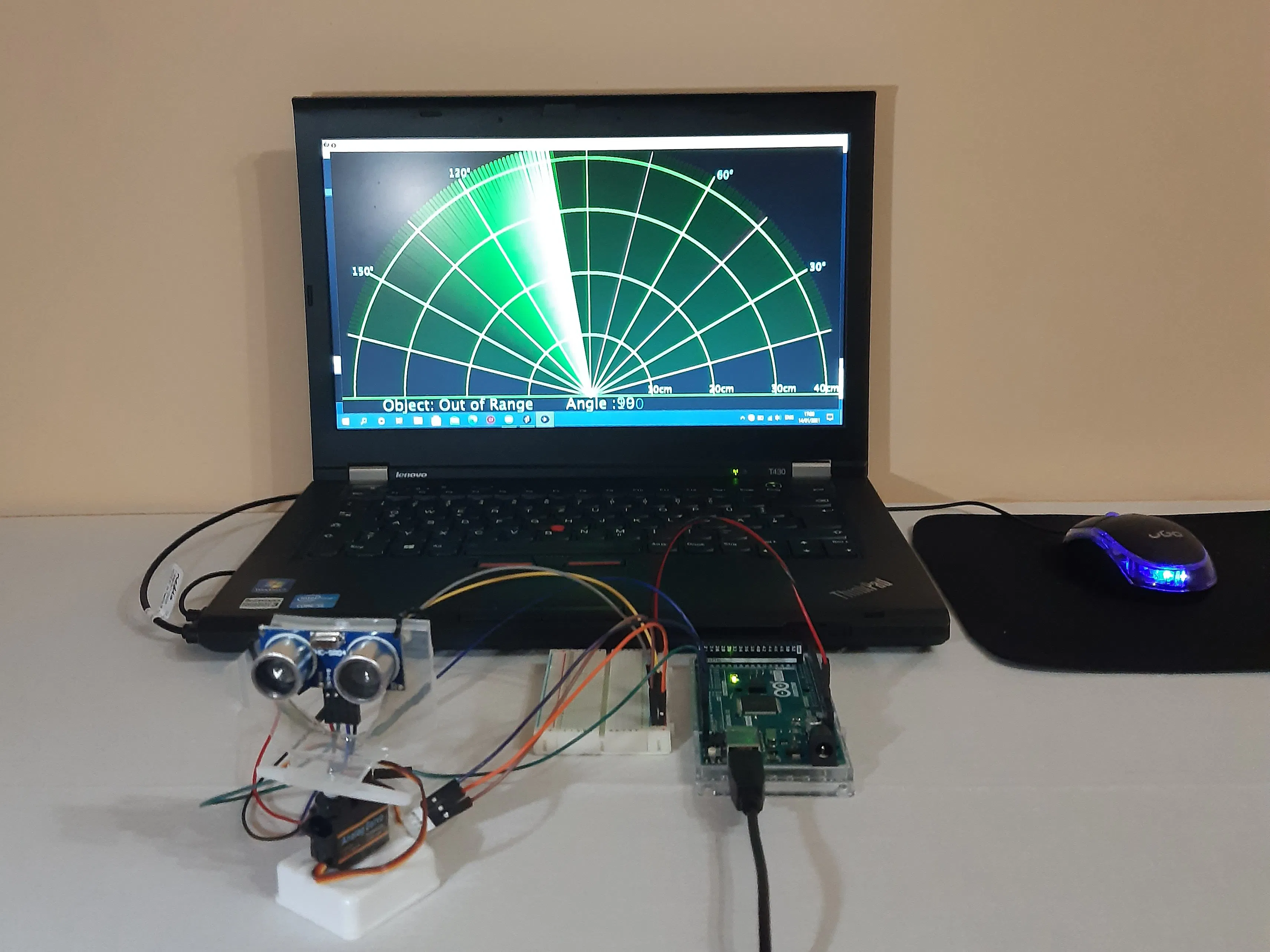## Things used in this project

### Hardware components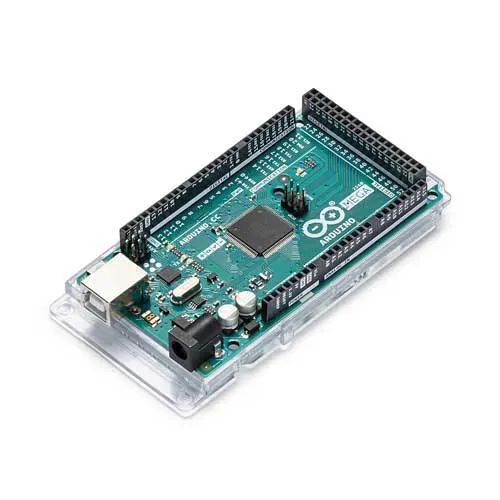Arduino Mega 2560
×1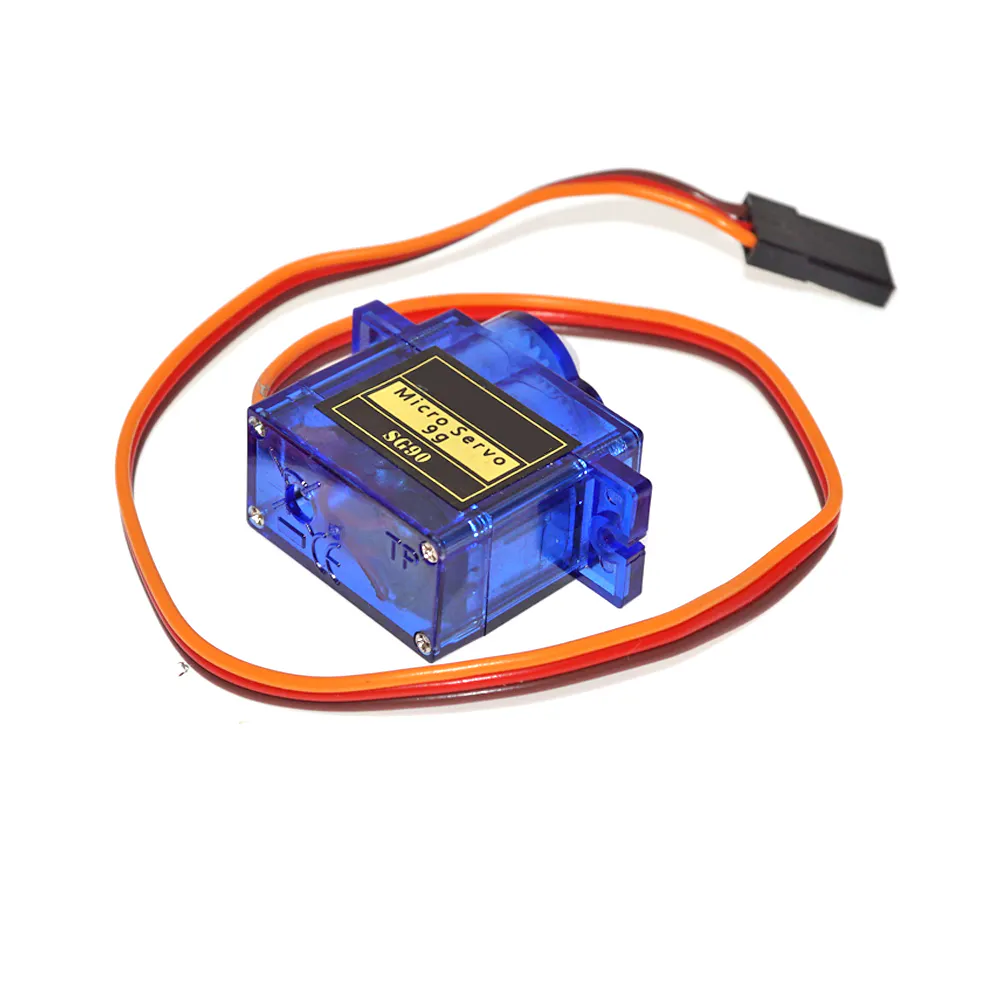SG90 Micro-servo motor
×1Ultrasonic Sensor - HC-SR04 (Generic)
×1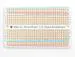Perma-Proto Breadboard Half Size
×1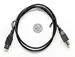USB-A to B Cable
×1
 Amazon Web Services Holder for Ultrasonic Sensor HC-SR04
×1

### Software apps and online services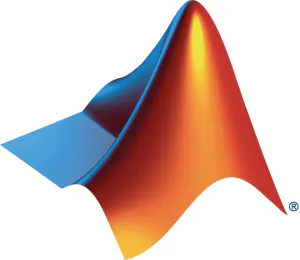MATLAB
 MATLAB Simulink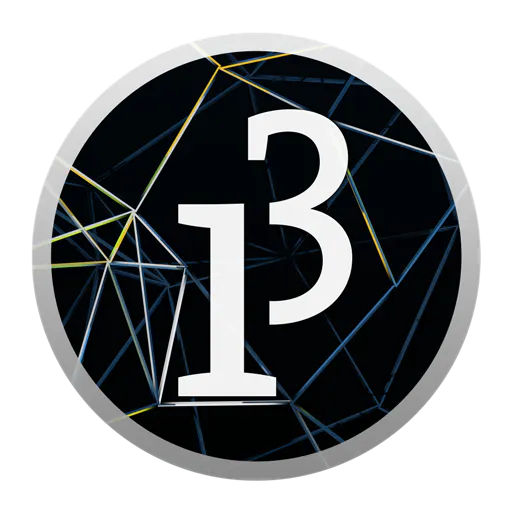The Processing Foundation Processing

Read more

## Schematics

### SCHEMATICS

Arduino Mega 2560 Sketch of connecting the components.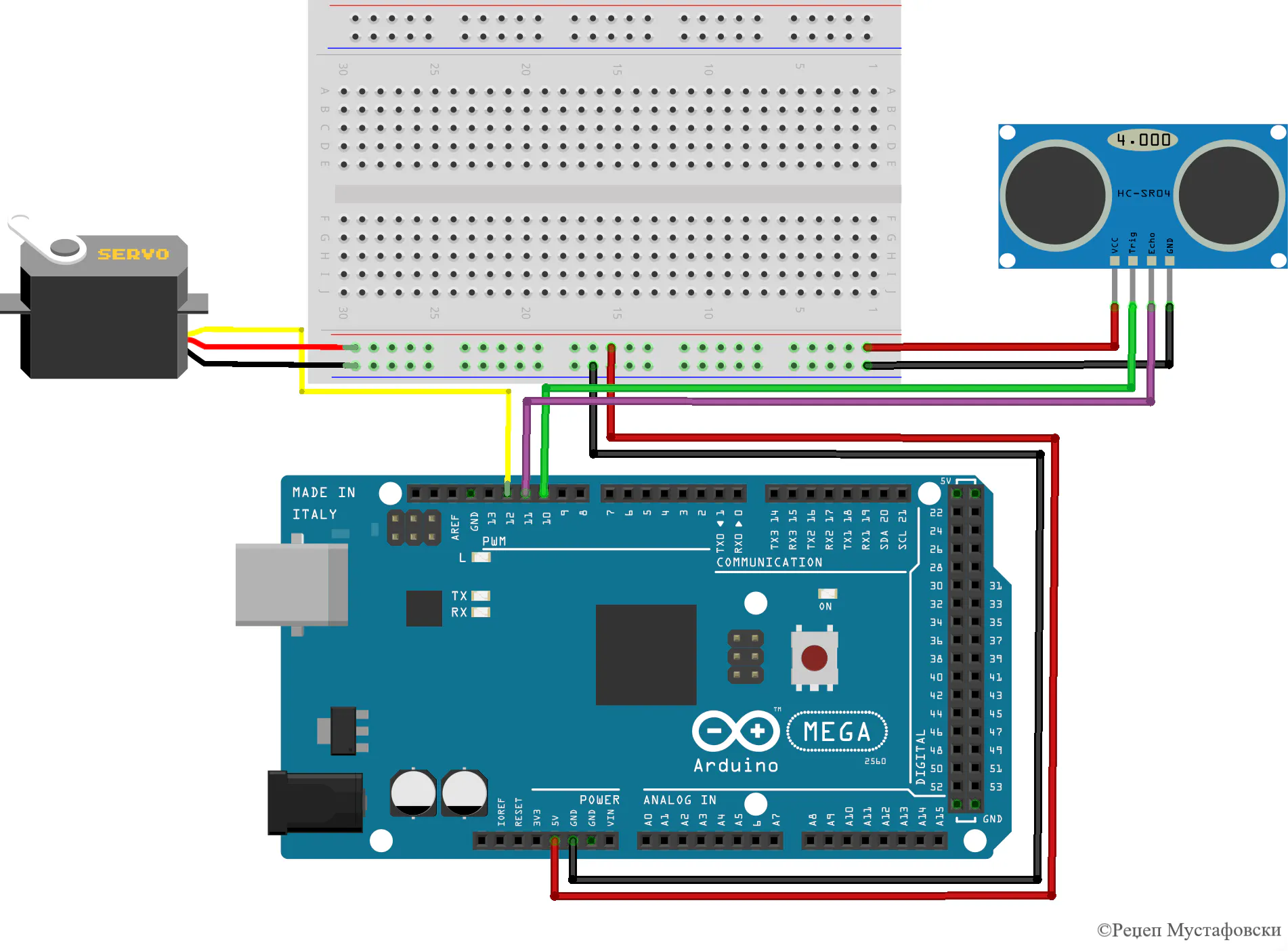### SCHEMATICS

Arduino Mega 2560 Sketch of connecting the components

## Code

### Simulink model in Matlab

Scheme
Appearance of the model in the Simulink simulation tool.
`No preview (download only).`

### Processing code

C/C++
Copy and paste the code in Processing.
```/*   Arduino Radar Project
*
*   Updated version. Fits any screen resolution!
*   Just change the values in the size() function,
*   with your screen resolution.
*
*  by Dejan Nedelkovski, updated by Rexhep Mustafovski
*
*/
import processing.serial.*; // imports library for serial communication
import java.awt.event.KeyEvent; // imports library for reading the data from the serial port
import java.io.IOException;
Serial myPort; // defines Object Serial
// defubes variables
String angle="";
String distance="";
String data="";
String noObject;
float pixsDistance;
float Razmer;  //
int iAngle, iDistance;
int index1=0;
int index2=0;
int pravec=1;
PFont orcFont;
void setup() {

fullScreen();   //

Razmer = (height-height*0.21)*0.025; //

smooth();
myPort = new Serial(this,"COM3", 115200); // starts the serial communication
myPort.bufferUntil('.'); // reads the data from the serial port up to the character '.'. So actually it reads this: angle,distance.
orcFont = loadFont("OCRAExtended-30.vlw");

}
void draw() {

fill(98,245,31);
textFont(orcFont);
// simulating motion blur and slow fade of the moving line
noStroke();
fill(0,5);
rect(0, 0, width, height-height*0.065);

fill(98,245,31); // green color
// calls the functions for drawing the radar
drawRadar();
drawLine();
drawObject();
drawText();

}
void serialEvent (Serial myPort) { // starts reading data from the Serial Port
// reads the data from the Serial Port up to the character '.' and puts it into the String variable "data".
data = myPort.readStringUntil('.');
data = data.substring(0,data.length()-1);

index1 = data.indexOf(","); // find the character ',' and puts it into the variable "index1"

angle= trim(data.substring(0, index1));                     // read the data from position "0" to position of the variable index1 or thats the value of the angle the Arduino Board sent into the Serial Port
distance= trim(data.substring(index1+1, data.length()));    // read the data from position "index1" to the end of the data pr thats the value of the distance
// new updated trimed data

// converts the String variables into Integer
iAngle = int(angle);
iDistance = int(distance);
}
void drawRadar() {
pushMatrix();
translate(width/2,height-height*0.074); // moves the starting coordinats to new location
noFill();
strokeWeight(2);
stroke(98,245,31);
// draws the arc lines

arc(0,0,2*40*Razmer,2*40*Razmer,PI,TWO_PI);     //      New updated formulas
arc(0,0,2*30*Razmer,2*30*Razmer,PI,TWO_PI);
arc(0,0,2*20*Razmer,2*20*Razmer,PI,TWO_PI);
arc(0,0,2*10*Razmer,2*10*Razmer,PI,TWO_PI);

// draws the angle lines
line(-width/2,0,width/2,0);
line(0,0,(-width/2)*cos(radians(30)),(-width/2)*sin(radians(30)));
line(0,0,(-width/2)*cos(radians(60)),(-width/2)*sin(radians(60)));
line(0,0,(-width/2)*cos(radians(90)),(-width/2)*sin(radians(90)));
line(0,0,(-width/2)*cos(radians(120)),(-width/2)*sin(radians(120)));
line(0,0,(-width/2)*cos(radians(150)),(-width/2)*sin(radians(150)));
line((-width/2)*cos(radians(30)),0,width/2,0);
popMatrix();
}
void drawObject() {
pushMatrix();
translate(width/2,height-height*0.074); // moves the starting coordinats to new location
strokeWeight(9);
stroke(255,10,10); // red color

pixsDistance = iDistance*Razmer; // converts the distance from the sensor from cm to pixels
// limiting the range to 40 cms
if(iDistance<40){
// draws the object according to the angle and the distance

line(pixsDistance*cos(radians(iAngle)),-pixsDistance*sin(radians(iAngle)),40*Razmer*cos(radians(iAngle)),-40*Razmer*sin(radians(iAngle)));  // new updated line

popMatrix();
}
void drawLine() {
pushMatrix();
strokeWeight(9);
stroke(30,250,60);
translate(width/2,height-height*0.074); // moves the starting coordinats to new location
line(0,0,40*Razmer*cos(radians(iAngle)),-40*Razmer*sin(radians(iAngle))); // new updated line
popMatrix();
}
void drawText() { // draws the texts on the screen

pushMatrix();
if(iDistance>40) {
noObject = "Out of Range";
}
else {
noObject = "In Range";
}
fill(0,0,0);
noStroke();
rect(0, height-height*0.0648, width, height);
fill(98,245,31);
textSize(25);

text("10cm",width/2+7*Razmer,height-height*0.0833);
text("20cm",width/2+17*Razmer,height-height*0.0833);
text("30cm",width/2+27*Razmer,height-height*0.0833);
text("40cm",width/2+37*Razmer,height-height*0.0833);

textSize(40);
text("Object: " + noObject, width-width*0.875, height-height*0.0277);
text("Angle: " + iAngle +" ", width-width*0.48, height-height*0.0277);
text("Distance: ", width-width*0.26, height-height*0.0277);
if(iDistance<40) {
text("        " + iDistance +" cm", width-width*0.225, height-height*0.0277);
}
textSize(25);
fill(98,245,60);
translate((width-width*0.4994)+width/2*cos(radians(30)),(height-height*0.0907)-width/2*sin(radians(30)));
rotate(-radians(-60));
text("30",0,0);
resetMatrix();
translate((width-width*0.503)+width/2*cos(radians(60)),(height-height*0.0888)-width/2*sin(radians(60)));
rotate(-radians(-30));
text("60",0,0);
resetMatrix();
translate((width-width*0.507)+width/2*cos(radians(90)),(height-height*0.0833)-width/2*sin(radians(90)));
rotate(radians(0));
text("90",0,0);
resetMatrix();
translate(width-width*0.513+width/2*cos(radians(120)),(height-height*0.07129)-width/2*sin(radians(120)));
rotate(radians(-30));
text("120",0,0);
resetMatrix();
translate((width-width*0.5104)+width/2*cos(radians(150)),(height-height*0.0574)-width/2*sin(radians(150)));
rotate(radians(-60));
text("150",0,0);
popMatrix();
}
```

## Credits

### rexhepmustafovski

0 projects • 3 followers